# Solution assignment 06 Optimizing f(x)

### Assignment 6

Find the extremes of the function: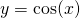on the interval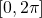. Verify whether they are maxima or minima by using the second derivative.

### Solution

The derivative of the function is: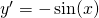The equation: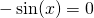has the following solutions on the given interval: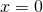,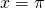orWe calculate the second derivative: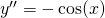Forwe have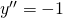and thus there is maximum in this point.

Forwe have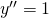and thus there is minimum in this point.

Voorwe haveand thus there is maximum in this point.

0